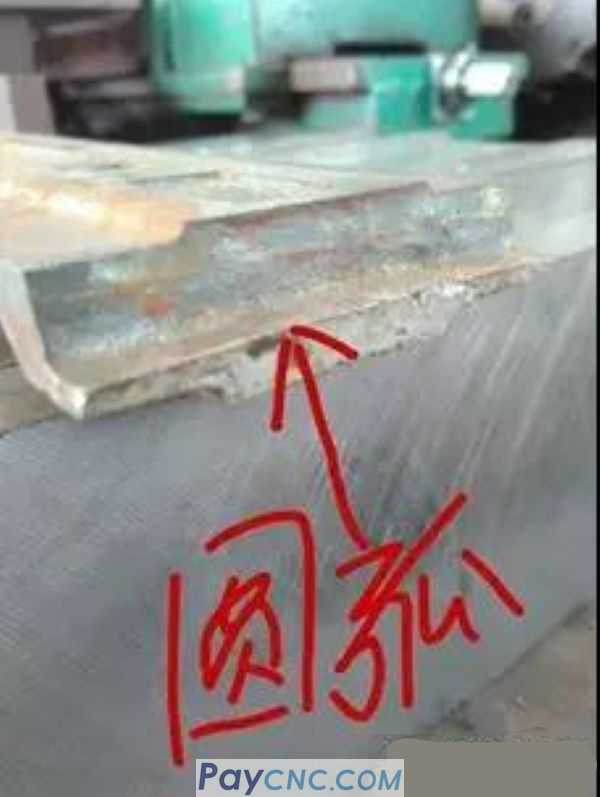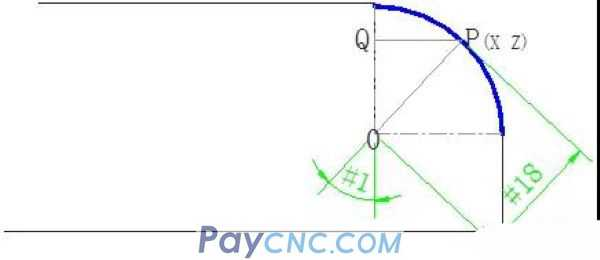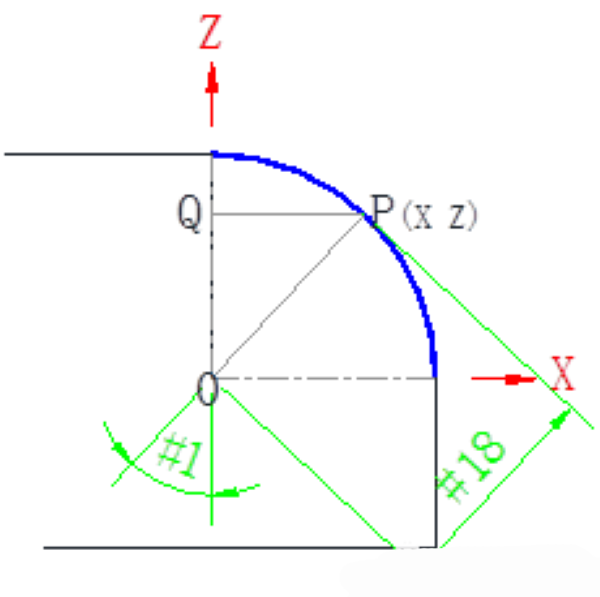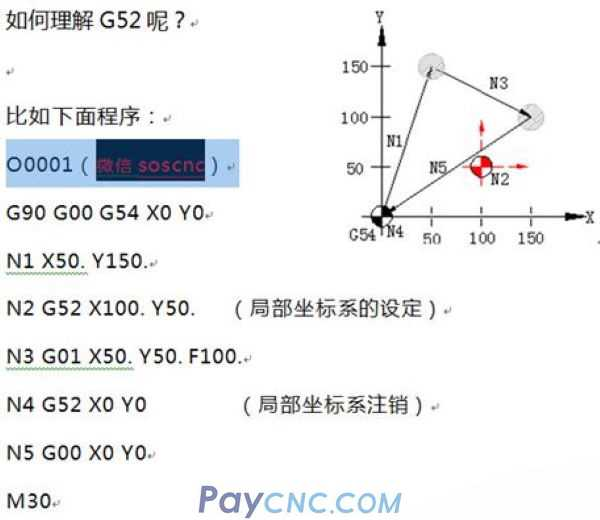A few days ago there was a master who wanted to process several batches of parts. The parts were not difficult. He just milled the plane and turned the edges into an arc by the way.but, There are several batches of these parts The arc size is not uniform Several special concave arc cutters of different sizes   With a standard end mill, the edges are chamfered by the way.       In fact, about using standard tools, writing general programs, chamfering arbitrary corners, and rounding corners       In fact, the simplest reason is that they did not understand the thought process behind these programming.   This is like doing a math problem. If you don't pay attention to the calculation process of arithmetic, even if you do more math problems and change the test questions you have done before, you may still not.   So I often say that cases are not important, because cases are everywhere, and it is more important to teach you the ideas and methods of writing programs.               There are more, not to list them all... They are all grounded thinking and practical methods...   E.g: Some time ago, a master Song, who had been working on counting cars, had been operating machine tools for several years. He had been able to program simple parts such as the outer circle of cars and boring. He had been unable to make breakthroughs in his skills. After dozens of days of work, he taught me the ideas of the tutorial. After realizing a little bit, he made a high-quality worm, and dumped a lot of colleagues of the same level around him. The master of 20 years praised him for his good work.           E.g: An apprentice doll who was still operating a CNC machine tool three years ago did not know any programming. According to the ideas and methods I taught, I learned step by step, and the opportunity came and I was promoted to a technician.           E.g: Some buddies, who have been operating for decades in the factory, have never had the opportunity to raise posts and raise salaries, improve their abilities, become stronger, and create opportunities... The road gets wider and wider...               For learning CNC programming, to thoroughly understand the programming ideas behind part programming, this is the core.   So, back to the beginning of the article, the problems encountered by the master:   To customize a concave arc tool with a special size, I want to use a general program to chamfer the edge with a standard end mill.   What are the ideas and methods for writing such parts?   In many cases, the essence of things is very simple, and programming is no exception, because whether it is software programming or manual programming, for a program, there are nothing more than two major components:   1. G command. 2. Point coordinates.   There are only dozens of CNC G commands commonly used, a few, but whether the part is a straight line, arc, or curved surface, it is composed of countless small points, and then the points are connected by small line segments. , Which constitutes a wide range of products.   So how to deal with countless point data? For example, if the edge of the above part is rounded to an arc R, the coordinates of the point on the arc must be calculated.   The arc is as follows:If point P in the above figure, it is considered any point on the arc. I constructed a right-angled triangle OPQ. The trigonometric function in mathematics stipulates that the relationship between sides and angles is as follows: SIN[#1]=QP/#18 COS[#1]=OQ/#18   How does the Pythagorean formula come from? There is no need to go into the mathematics. You only need to know the relationship between them and apply directly.   Then I directly apply the above formula to calculate the coordinate point corresponding to point P, as follows:   QP= SIN[#1]*#18 (The line segment QP represents the coordinate value of point P in the X direction) OQ= COS[#1] *#18 (the line segment OQ represents the coordinate value of point P in the Z direction)I then expressed OP and OQ in the above arithmetic formula with macro variables recognized by the machine tool, and then the following arithmetic formula was obtained. #24= SIN[#1]*#18 #26= COS[#1]*#18   OK, #24 and #26 are the coordinate points in the X direction and the Z direction of any point on the arc, but   It takes the center of the arc as the coordinate origin.   When the operator sets the tool, it is difficult to establish the origin of the workpiece on the center of the arc. For example, the figure below is set at the intersection of two straight edges of the workpiece. (Or elsewhere).How to do it? For example, you can use G52 to establish a local coordinate system.   Come on, here is an example of G52 usage   format: G52 X_ Y_ Z_ (Set local coordinate system) ………. G52 X0 Y0 Z0 (Cancel local coordinate system)Look at the above program carefully. Look carefully at the icon on the right. Is it simple? Very useful   OK, if the programming origin is the intersection of the two straight edges of the workpiece (yellow in the figure below)Then G52X-#18Z-#18, the local coordinate system is established in this way, and the above problem of using the arc center as the coordinate origin is solved.     Well, after analyzing this, the tool diameter is also considered in the program below, as shown in the following diagram: Set the tool diameter variable as: #20If the tool diameter #20 is considered in the program, #24 = SIN[#1]*#18 is the coordinate point in the X direction of the arc (as shown in the figure above), which needs to be offset by a tool radius value to the right, and finally #24= SIN[#1]*#18+#20/2     Well, I spent a lot of space to deduced the process of compiling the numerical control macro program to calculate the point. I hope to give you some inspiration and gains.   At this point, I went directly to the program: